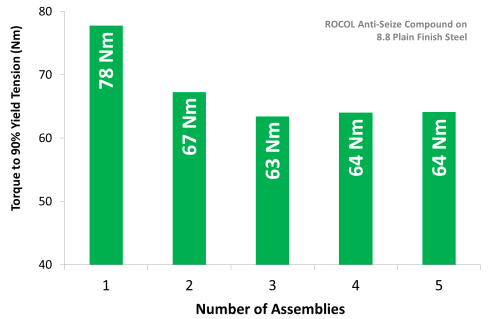03 October 2018

# A deeper understanding of Anti-Seize

It is suggested that the gasket manufacturer’s instructions are followed.1. Should Anti-Seize be applied on gaskets?

It is suggested that the gasket manufacturer’s instructions are followed.

In some cases, it is not recommended that anti-seize compounds are applied to gaskets because it may cause slippage of the gasket under load or distortion of the gasket, both of which may cause leakage.

However, in some systems, the use of anti-seize on gasketed joints is highly recommended because it can significantly ease disassembly in corrosive or extreme environments.

2. What is the Torsion-Tension Relationship?

Bolts, studs and fasteners are used to produce a clamping force that given stability to a jointed interface. This clamping force is produced by tensioning the fastener.

In most threaded fasteners, tension is applied by applying torque to the nut or bolt head. Therefore, it is necessary to know the relationship between the input torque and the resulting tension in the bolt. Some larger bolts are directly tensioned.

For many systems, bolt tightening charts report the input torque required to produce the correct bolt tension and clamping load.

However, many tightening charts are based on dry or lightly oiled fasteners i.e. without optimum lubrication. Therefore, when an anti-seize compound is applied, the original input torque will not produce the same tension.

Because quality anti-seize compounds are generally excellent lubricants, the required torque to produce the correct tension will be lower than for a dry fastener.

There are several ways to achieve the correct torque setting when an anti-seize is used. These include:
• Torque Reduction Factor / Friction Factor
• The K-Factor Method
• Using the Coefficient of Friction

3. What is Torque Reduction Factor / Friction Factor?

Because the use of an anti-seize changes the torsion-tension relationship of a fastener system, torque settings need to be recalculated.

The dry/oiled torque value can be multiplied by a Torque Reduction Factor to produce the correct torque value for the particular anti-seize compound and material combination. Thus, the correct tension can be achieved.

There are limitations to the accuracy of Torque Reduction Factors:
• The torque-tension relationship for a dry/oiled fastener is extremely variable. Therefore, any value derived relative to this will inherit the same wide variability.
• It is only valid for a particular set of materials and fastener geometry. A torque reduction factor is not universally applicable for an anti-seize under any condition.

Therefore, deriving the torque setting from a K-factor or Coefficient of Friction is simpler and more accurate. ROCOL would generally recommend producing torque tables using these methods. ROCOL provide data and formulae to achieve this, and are happy to give guidance

4. What is K-Factor?

Because the use of an anti-seize changes the torsion-tension relationship of a fastener system, torque settings need to be recalculated.

A more accurate alternative to the torque reduction factor, K-factor describes the fastener tension varies with input torque for a specific set of conditions. The input torque required to achieve the correct fastener tension can be calculated using the following equation:

T=K×F×D

Where:
T = Input Torque (Nm)
K = K-Factor
F = Tension (N)
D = Nominal Diameter of Fastener (m)

The K-Factor is measured under a specific set of conditions. Therefore, if the fastener material, thread type (coarse, fine) or assembly (washer, no washer, self-locking) is different to the conditions that the K-Factor was measured under, it may not be valid and care should be taken to ensure that the correct fastener tension is achieved.

ROCOL provide K-Factors for each anti-seize product with a range of common materials. This ensures that more accurate fastener tensions can be achieved via this calculation method. The data shows that K-Factor can vary widely with fastener material.

K-Factors cannot be used to compensate for changes in thread type (coarse, fine etc.). In order to do so, the friction coefficient of the anti-seize compound should be used

5. What is the Friction Coefficient of an Anti-Seize Compound?

Because the use of an anti-seize changes the torsion-tension relationship of a fastener system, torque settings need to be recalculated.

The most accurate way to calculate the input torque that will produce the correct tension in the bolt is using the friction coefficient (μ). Whilst this is more complicated and intensive that the K-Factor equation, it is more accurate and allows variations in thread geometry to be taken into account.

ROCOL report coefficients of friction for a range of common fastener materials. Therefore, using this method, the fastener material, anti-seize compound and thread geometry can be considered. However, care should still be taken with alternative bolt geometries (oversized washers, self-locking nuts) and if the bolt will be re-used (because the friction coefficient can change with re-use).

Several equations can be used to calculate the input torque required to achieve the correct tension in a fastener. ROCOL calculate coefficient of friction values from the following:

T=F×[(0.159×P)+(0.577×d×μ)+(D_f×μ/2)]

Where T = Torque Applied (Nm)
F = Tension Generated in Fastener (N)